• 复化辛普森公式python
2021-12-29 22:34:13

# 数值积分

##### 调包
import math
import numpy as np
import matplotlib.pyplot as plt


fStr为被积函数函数名（str）

### 梯形求积

#梯形求积
def Trapezium(a,b,fStr):
return (b - a) / 2 * (globals()[fStr](a) +  globals()[fStr](b))


### 复化梯形

#复化梯形
def TrapeziumComplex(a,b,epsilon,fStr):
def F(x):
return globals()[fStr](x)
n = 0
h0 = b - a
T0 = h0 / 2 * (F(a) + F(b))
while True:
n += 1
h1 = h0 / 2
m = 2**(n - 1)
sum = 0
for k in range(1,m + 1):
sum += F(a + (2 * k - 1) * h1)
T1 = T0 / 2 + h1 * sum
if abs(T1 - T0) < epsilon:
break
T0 = T1
h0 = h1
return T1,n


### 辛普森求积

#辛普森求积
def Simpson(a,b,fStr):
return (b - a) / 6 * (globals()[fStr](a) +  globals()[fStr](b) + 4 * globals()[fStr]((a + b) / 2))


### 复化辛普森

#复化辛普森
def SimpsonComplex(a,b,epsilon,fStr):
def F(x):
return globals()[fStr](x)
n = 0
h0 = (b - a) / 2
S0 = h0 / 3 * (F(a) + 4 * F((a + b) / 2) + F(b))
while True:
n += 1
h1 = h0 / 2
m = 2**(n - 1)
sum1 = 0
sum2 = 0
for k in range(1,m * 2 + 1):
sum1 += F(a + (2 * k - 1) * h1)
if k <= m:
sum2 += F(a + (4 * k - 2) * h1)
S1 = S0 / 2 + h1 / 3 * (4 * sum1 - 2 * sum2)
if abs(S1 - S0) < epsilon:
break
S0 = S1
h0 = h1
return S1,n


### 龙贝格求积

#龙贝格算法
def Romberg(a,b,epsilon,fStr):
def F(x):
return globals()[fStr](x)
# 存放求积分范围
h = b - a
# 用于存放 T S C R  的计算结果
T = [[j for j in [0, 0, 0, 0]] for i in range(4)]
# 用于计行数
i = 1
T_1 = h * (F(b) + F(a)) / 2
T = T_1
T = T_1 / 2 + F((b - a) / 2) * h / 2
T = (T * 4 ** i - T) / (4 ** i - 1)
# 精度达不到设定值时继续执行
# 当行数小于四时，根据行数选择计算 T S C R 中的哪几个
while abs(T[i][i]-T[i][i-1]) > epsilon:
i += 1
sum = 0
if i == 4 :
break
for j in range(2 ** (i - 1)):
sum += F(a + (2 * j + 1) * h / 2 ** i)
T[i] = T[i - 1] / 2 + sum * h / 2 ** i
for j in range(1, i + 1):
T[i][j] = (T[i][j - 1] * 4 ** i - T[i - 1][j - 1]) / (4 ** i - 1)
# 当行数大于四时，计算全部的 T S C R
while abs(T[i-1][-1] - T[i-1][-2]) > epsilon:
sum = 0
T.append([])
for j in range(2 ** (i - 1)):
sum += F(a + (2 * j + 1) * h / 2 ** i)
T[i].append(T[i - 1] / 2 + sum * h / 2 ** i)
for j in range(1, 4):
T[i].append((T[i][j - 1] * 4 ** i - T[i - 1][j - 1]) / (4 ** i - 1))
i += 1
return T[-1][-1],i


### 自适应求积

#自适应求积
def F(x):
return globals()[fStr](x)
h = (a + b) / 2
ST = Simpson(a,b,fStr)
SL = Simpson(a,h,fStr)
SR = Simpson(h,b,fStr)
if(abs(SL + SR - ST) <= 15.0 * epsilon):
return SL + SR + (SL + SR - ST) / 15.0算法
更多相关内容
• 自编Python程序实现数值计算矩形区域二重积分，使用复化辛普森法。以函数f=xsiny在0和pi/2区域上的积分为例。网格节点数m，n需为2的倍数。对于非矩形区域可以使用虚拟节点和区域，填补为矩形区域后计算，填补区域上...
• Python实现梯形公式 、辛普森公式复化梯形公式、复化辛普森公式 数值求积公式概念 梯形公式与辛普森公式 梯形公式与辛普森公式的余项 复化求积公式 复化梯形公式与其余项 复化辛普森公式与其余项 Python实现...

数值分析：梯形公式 、辛普森公式、复化梯形公式、复化辛普森公式

# Python实现梯形公式 、辛普森公式、复化梯形公式、复化辛普森公式

## 数值求积公式概念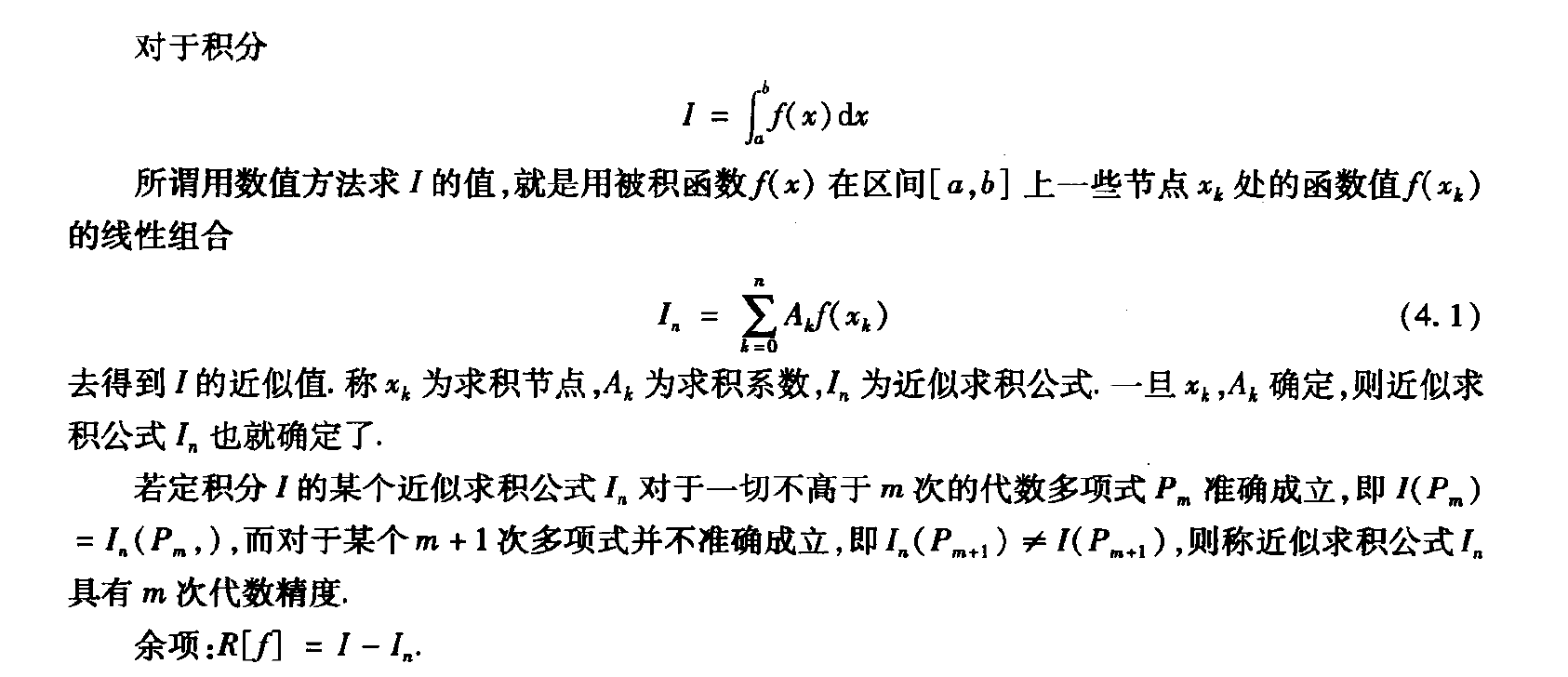## 梯形公式与辛普森公式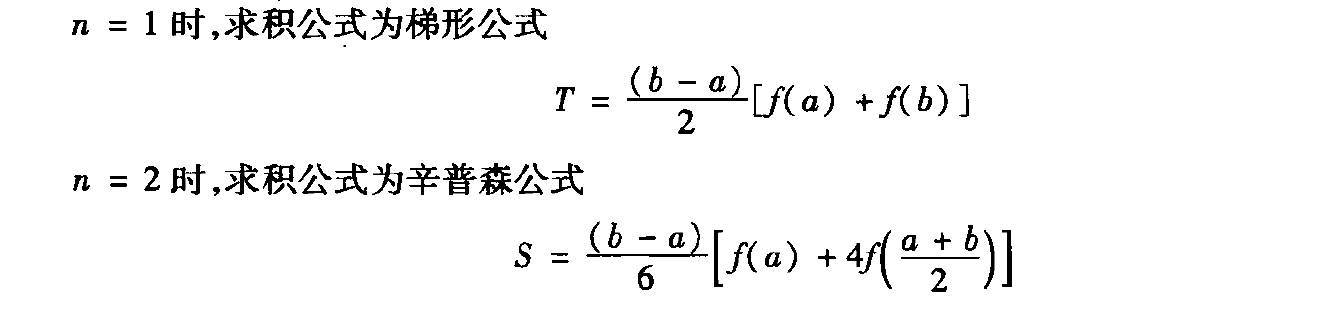## 梯形公式与辛普森公式的余项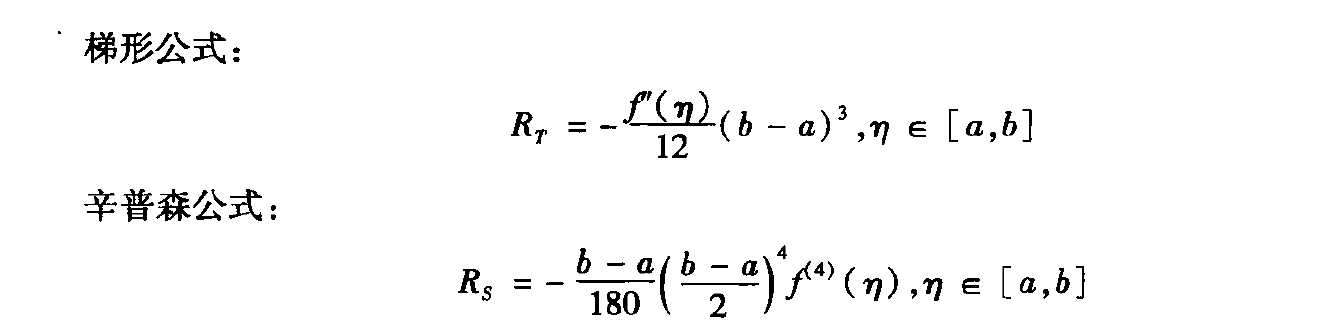## 复化求积公式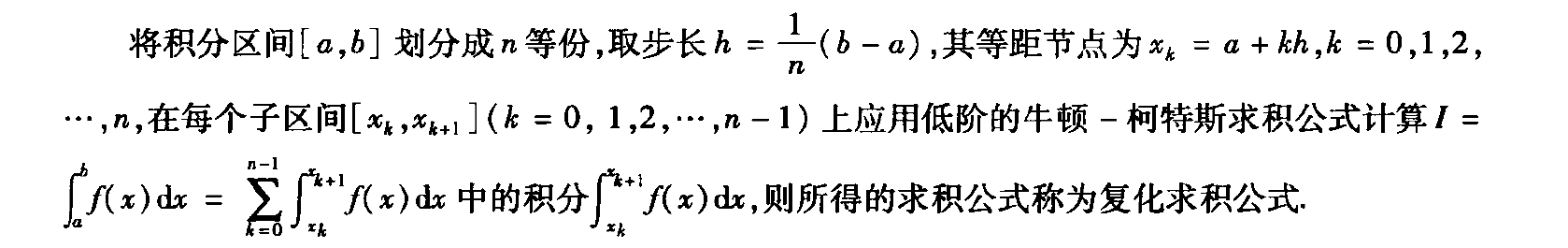### 复化梯形公式与其余项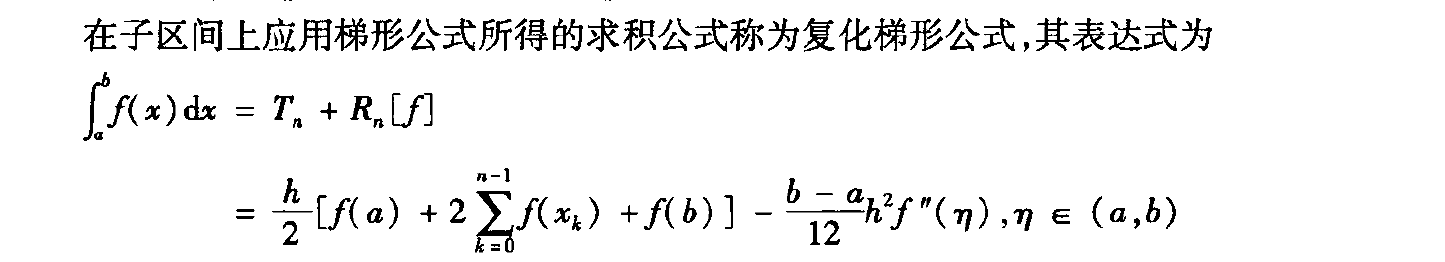### 复化辛普森公式与其余项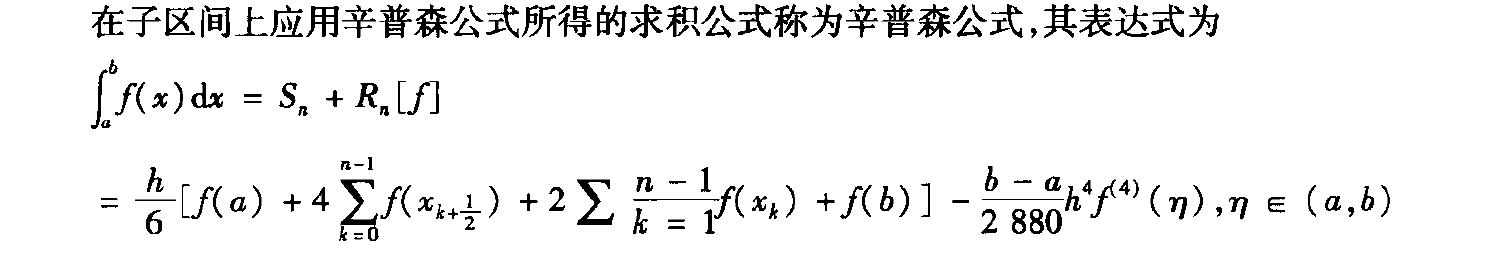### Python实现四种公式.

#### 题目.

Python编写梯形公式、辛普森公式、复化梯形公式、复化辛普森公式
并利用其分别求解sqrt(x) * log(x) 与 sin(x)/x 在(0,1)上的积分。

具体代码实现：

import math
import numpy as np
import matplotlib.pyplot as plt
#待求解数值积分sqrt(x) * log(x)
def f1(x):
if (float(np.fabs(x))<1e-15) :
return 0
y=np.sqrt(x) * np.log(x)
return y
#待求解数值积分sin(x)/x
def f2(x):
if (float(np.fabs(x)) < 1e-15):
return 1
y=np.sin(x)/x
return y
#梯形公式 f为待求解积分 a为积分下限 b为积分上限
def TX(f,a,b):
TX = 0.5 * (b - a) * (f(a) + f(b))
print("梯形公式计算结果为：TX = ", TX)
#辛普森公式 f为待求解积分 a为积分下限 b为积分上限
def XPS(f,a,b):
XPS = (b-a)*(f(a)+4*f((a+b)/2)+f(b))/6.0
print("辛普森公式计算结果为：XPS = ", XPS)
#复化梯形公式 f为待求解积分 a为积分下限 b为积分上限 n为区间等分数
def FHTx(f,a,b,n):
ti=0.0
h=(b-a)/n
ti=f(a)+f(b)
for k in range(1,int(n)):
xk=a+k*h
ti = ti + 2 * f(xk)
FHTx = ti*h/2
print("复化梯形公式计算结果为：FHTx = ", FHTx)
#复化辛普森公式 f为待求解积分 a为积分下限 b为积分上限 n为区间等分数
def FHXPs(f,a,b,n):
si=0.0
h = (b - a) / (2 * n)
si=f(a)+f(b)
for k in range(1,int(n)):
xk = a + k * 2 * h
si = si + 2 * f(xk)
for k in range(int(n)):
xk = a + (k * 2 + 1) * h
si = si + 4 * f(xk)
FHXPs = si*h/3
print("复化辛普森公式计算结果为：FHXPs = ", FHXPs)

def main():
a = input("a = ")  # 积分下限
b = input("b = ")  # 积分上限
a = float(a)  # 强制转换为float类型
b = float(b)
n = input("n = ") #将区间分成为n等份
n = float(n)
#TX(f2,a,b) #调用梯形公式求解
#XPS(f2,a,b) #调用辛普森公式求解
#FHTx(f2,a,b,n) #调用复化梯形公式求解
FHXPs(f2,a,b,n) #调用复化辛普森公式求解

if __name__ == '__main__':
main()

展开全文算法
• 这里并不能够说完全实现了复化辛普生公式，因为这里面涉及到具体的函数，而我们需要事先知道函数表达式，才能够求出来。 故而这里是以书上的一道例题来写 例题以及书上结果如下： 这里我也并没有按照流程图来写...

这里并不能够说完全实现了复化辛普生公式，因为这里面涉及到具体的函数，而我们需要事先知道函数表达式，才能够求出来。
故而这里是以书上的一道例题来写

例题以及书上结果如下：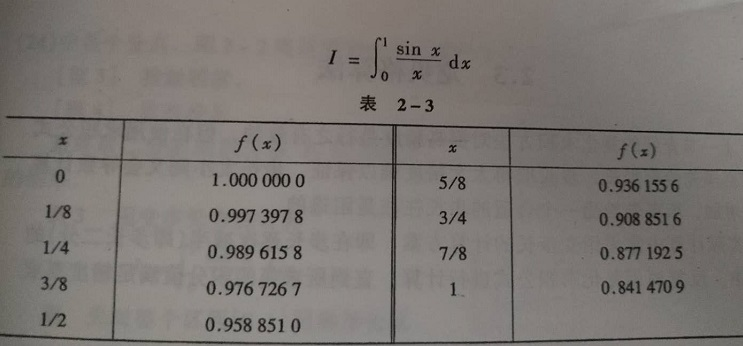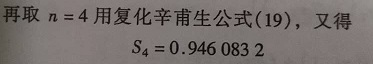这里我也并没有按照流程图来写（不过大同小异），而是按照表达式来写
流程图和表达式如下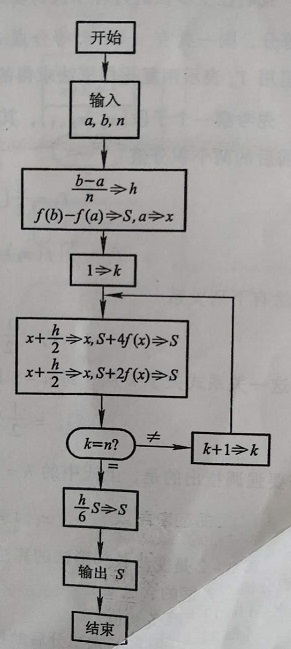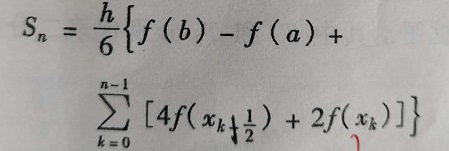代码如下：

# coding=gbk;
#因为使用复化辛普生公式会涉及到函数的具体形式
# 所以这里我就暂且令 f(x)=sin(x)/x
import math;
def compute_fx(temp):  #用来计算函数值
if temp==0:
return 1.0;
return math.sin(temp)/temp;

def fuhua_simpson(a1,b1,h1,n1):  #复化辛普生
S=compute_fx(b1)-compute_fx(a1);
account=0;
x=a1;
while account<n:
S+=4*compute_fx(x+h1/2)+2*compute_fx(x);
x+=h;
account+=1;
return S;

list_temp=input("请分别输入积分得上下限以及想要将其几等分：").split(" ");
a=float(list_temp);
b=float(list_temp);
n=float(list_temp);
h=(b-a)/n; #h是步长
result=fuhua_simpson(a, b, h, n);
result=result*h/6;
print(result);


运行结果：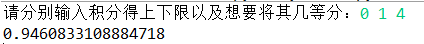遇事不决，可问春风

展开全文计算机数值分析
• 基于复合梯形公式和复合辛普森求积公式计算积分在python中的实现.txt
• #复合辛普森 def xps(a,b,n): h=(b-a)/n x=a s=fun(x)-fun(b) for k in range(1,n+1): x=x+h/2 s=s+4*fun(x) x=x+h/2 s=s+2*fun(x) result=(h/6)*s return result a=3 b=6 n=9 t=tx(a,b,n) p=xps(a,b,n) ...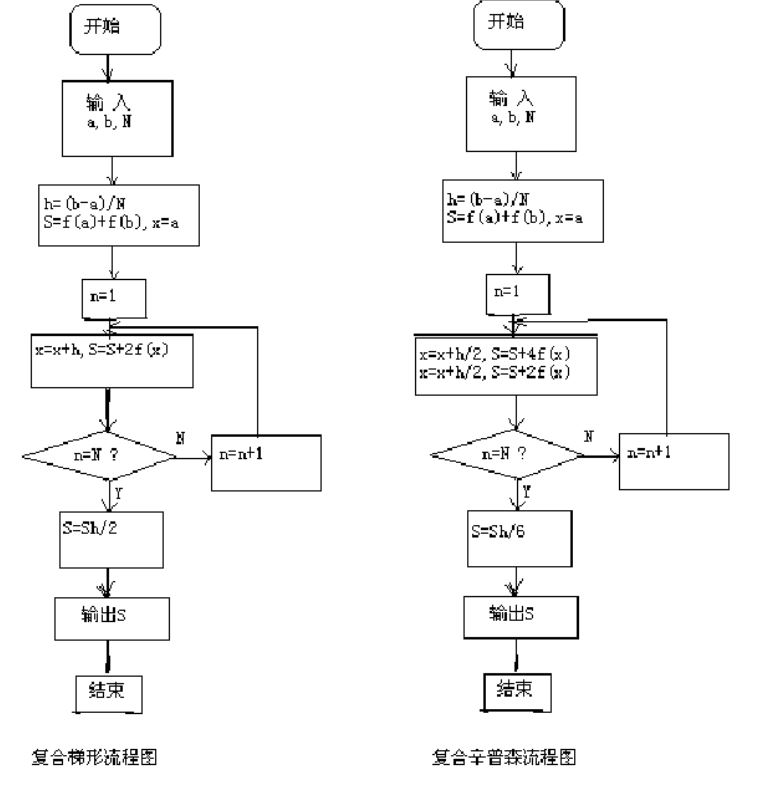#被积函数
def fun(x):
return x/(4+x*x)
#复合梯形
def tx(a,b,n):
h=(b-a)/n
x=a
s=fun(x)-fun(b)
for k in range(1,n+1):
x=x+h
s=s+2*fun(x)
result=(h/2)*s
return result
#复合辛普森
def xps(a,b,n):
h=(b-a)/n
x=a
s=fun(x)-fun(b)
for k in range(1,n+1):
x=x+h/2
s=s+4*fun(x)
x=x+h/2
s=s+2*fun(x)
result=(h/6)*s
return result
a=3
b=6
n=9
t=tx(a,b,n)
p=xps(a,b,n)
print(t,p)
0.5620542501164288 0.5619649373692952
代码求的是函数x/(4+x*x)在x=3到6上的积分
展开全文数值积分
• 2.复合辛普森公式 python实现 import math def fun(x): return math.sin(x)/(x+1e-16) #加上1e-16避免除零错误 # 复合梯度 def tx(a,b,n): h=(b-a)/n fxi=0 xi=a for i in range(1,n): xi=xi+h...
• python代码 import numpy as np a, b = input("积分区间：").split(' ') n = int(input("子区间个数：")) a = int(a) b = int(b) x_i = np.linspace(a, b, n+1) # 区间结点 h = (b-a)/n # 原函数 def f(x): ...
• 分别使用复合梯形公式和复合辛普森公式计算如下积分： ∫262x4+x2dx  \int_{2}^{6} \frac{2x}{4+x^2}dx\, ∫26​4+x22x​dx 并于该积分的准确值进行比较。注意，采用复合梯形公式和复合辛普森公式时，所使用的等分...数学
• 用程序来求积分的方法有很多，这篇文章主要是有关牛顿-科特斯公式。学过插值算法的同学最容易想到的就是用插值函数代替被积分函数来求积分，但实际上在大部分场景下这是行不通的。插值函数一般是一个不超过n次的...
• 复合梯形公式 将区间[a,b][a,b][a,b] 划分 nnn 等分，分点 xk=a+khx_k = a+khxk​=a+kh ，h=b−anh=\frac{b-a}{n}h=nb−a​ ，k=0,1,...,nk=0,1,...,nk=0,1,...,n，在每个子区间[xk,xk+1]][x_k,x_k+1]][xk​,xk​+1]...算法
• 实验目的或要求1、利用复化梯形公式复化simpson公式计算积分2、比较计算误差与实际误差实验原理(算法流程图或者含注释的源代码)取n=2,3,…,10分别利用复化梯形公式复化simpson公式计算积分120Ixdx，并与真值...
• 1.求积公式余项 1.1 定义 R[f]=∫ab ⁣ ⁣ ⁣f(x)dx−∑k=0nAkf(xk)=Kf(m+1)(η),(1)R[f]=\int_a^b\!\!\!f(x)\mathrm{d}x-\sum_{k=0}^nA_kf(x_k)=Kf^{(m+1)}(\eta ),(1)R[f]=∫ab​f(x)dx−k=0∑n​Ak​f(xk​)=...算法
• 问题 求 Python代码 from numpy import exp def f(x): """被积函数e^(x^2)"...辛普森公式""" return (b-a)*(f(a)+4*f((a+b)/2)+f(b))/6 def cotes(a,b): """数值积分 Cotes公式
• Python实现复合辛普森求积公式# -*-..."""simpr函数为用复合辛普森公式求积分 f是被积函数 a,b分别为积分的上下限 n是子区间的个数 s是梯形总面积，即所求积分数值""" h = (b - a) / (2 * n) s1 = 0 s2 = 0 for k i
• 最近利用碎片时间在读Allen B.Downey的《贝叶斯思维：统计建模的Python学习法》，顺便用手机上的Pythonista写实例。因为Pythonista没有scipy科学计算包，遇上需求标准正态累积分布函数的时候就只能抓瞎，为此决定...
• 这里以函数y=1举例。 ∫011dx=x∣01=1−0=1\int_0^1 1\mathrm{d}x = x|_0^1 = 1 - 0 = 1∫01​1dx=x∣01​=1−0=1 代码如下： num = 101 x_min = 0.0 x_max = 1.0 x = np.linspace(x_min, x_max, num) ...
• 欧拉公式求长期率的matlab代码MATH 210数学计算入门 的MATH 210是Python数学计算的简介。 我们从基本的Python编程开始，包括数据类型，...辛普森规则的误差公式 阅读周 2月26日 评论：梯形法则，辛普森法则和QUADPACK
• 主要介绍了python实现数值积分的Simpson方法,实例分析了Python实现积分运算的相关技巧,需要的朋友可以参考下
• 复化梯形公式 将区间n等分，每个小区间分别用梯形公式（两个节点）求积分，化简得到如上公式。 /** *@name Cotes：复化梯形公式 *@param1 below：区间下限 *@param2 upper：区间上限 *@param3 n：划分子区间的......